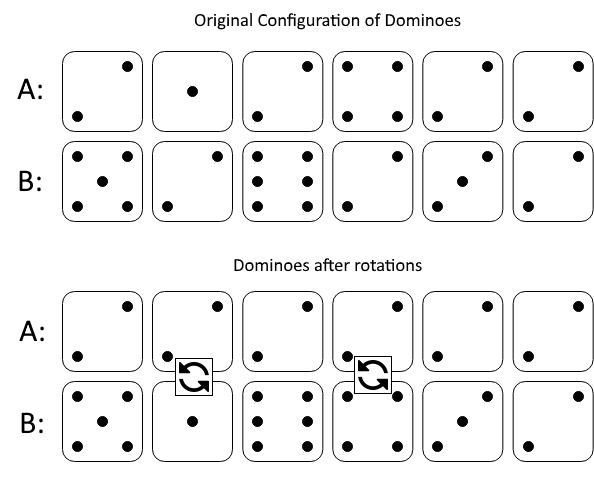# 1007. Minimum Domino Rotations For Equal Row 行相等的最少多米诺旋转

@TOC

## # 题目描述

In a row of dominoes, `A[i]` and `B[i]` represent the top and bottom halves of the `i`-th domino. (A domino is a tile with two numbers from 1 to 6 - one on each half of the tile.)

We may rotate the `i`-th domino, so that `A[i]` and `B[i]` swap values.

Return the minimum number of rotations so that all the values in `A` are the same, or all the values in `B` are the same.

If it cannot be done, return `-1`.

Example 1:``````Input: A = [2,1,2,4,2,2], B = [5,2,6,2,3,2]
Output: 2
Explanation:
The first figure represents the dominoes as given by A and B: before we do any rotations.
If we rotate the second and fourth dominoes, we can make every value in the top row equal to 2, as indicated by the second figure.
``````

Example 2:

``````Input: A = [3,5,1,2,3], B = [3,6,3,3,4]
Output: -1
Explanation:
In this case, it is not possible to rotate the dominoes to make one row of values equal.
``````

Note:

1. 1 <= A[i], B[i] <= 6
2. 2 <= A.length == B.length <= 20000

## # 解题方法

### # 遍历一遍

1.如果出现最多的数字的次数<长度，无论第一第二都不行。 2.如果出现最多的次数=长度，这个时候第二多次数如果等于N，那么两者效果一样，如果第二多次数如果小于N，那么不可能。 3.如果出现最多的次数>N，这个时候一定会出现某些牌的正反面都是该最多的数字。此时第二多的数字没有机会。

Python代码如下：

``````class Solution(object):
def minDominoRotations(self, A, B):
"""
:type A: List[int]
:type B: List[int]
:rtype: int
"""
N = len(A)
count = collections.Counter(A + B)
if count.most_common(1) < N:
return -1
target = count.most_common(1)
a_swap = 0
b_swap = 0
for i in range(N):
if A[i] == B[i]:
if A[i] == target:
continue
else:
return -1
elif A[i] == target:
b_swap += 1
elif B[i] == target:
a_swap += 1
else:
return -1
return min(a_swap, b_swap)
``````

## # 日期

2019 年 3 月 10 日 —— 周赛进了第一页！